## Abstract

Load forecasts of short lead times ranging from an hour to a day ahead are essential for improving the economic efficiency and reliability of power systems. This paper proposes a hybrid model based on the wavelet transform (WT) and the weighted nearest neighbor (WNN) techniques to predict the day ahead electrical load. The WT is used to decompose the load series into deterministic series and fluctuation series that reflect the changing dynamics of data. The two subseries are then separately forecast using appropriately fitted WNN models. The final forecast is obtained by composing the predicted results of each subseries. The hourly electrical load of California and Spanish energy markets are taken as experimental data and the mean absolute percentage error (MAPE), Weekly MAPE (WMAPE) and Monthly MAPE (MMAPE) are computed to evaluate the forecasting performance of the next-day load forecasts. The forecasting efficiency of the proposed model is evaluated using db2, db4, db5 and bior 3.1 wavelets. The results demonstrate the forecasting accuracy of the proposed hybrid model.

## Introduction

Load forecasting in the current, increasingly liberalized electricity power markets is of crucial importance as a means for producers to optimize and rationalize energy supply . Short-term load forecasts with lead times ranging from an hour to several days ahead are essential for improving the economic efficiency and raising the reliability of power system operations . This importance has led to the development of a wide variety of models/techniques differing in complexity, flexibility and data requirement . Forecasting models for load forecasting can be broadly classified into conventional (statistical), intelligent, and hybrid models. Surveys over models and methods for load forecasting can be found in [4-8]. The conventional models are linear and are known to show some weakness in the presence of special events and nonlinearity [9, 10]. Intelligent models designed using artificial intelligence techniques are found to be particularly useful in modeling the uncertain and nonlinear patterns within the load data. Among all intelligent techniques, Artificial Neural Network (ANN) models are the most popular, as they are able to give better performance in dealing with the nonlinear relationships among the input variables [6, 11]. However, in spite of the wide-spread use of neural networks, the technique suffers from a number of limitations, including difficulty in determining the optimum network topology and training parameters .

The efficiency of these methods is usually dependent on current tuning of their adjustable parameter, for example, the number of hidden models of the nearest neighbor (NN) . This has led to the development of hybrid/combination models that blend different techniques to enhance the performance and overcome the limitations of existing models . The basic idea of combining different models in forecasting is to use each models unique feature to capture different patterns within the data, thereby enhancing the accuracy of the forecasts . Both theoretical and empirical findings in the literature show that combining different methods is an effective and efficient way to improve forecasts . Hybrid models that combine the wavelet transform (WT) with other techniques have been extensively used for short-term load forecasting (STLF) . The WT is known to provide a sound mathematical technique for designing and deploying filters, which facilitate interpretation, understanding the data and the analysis methodology . WT has been successfully integrated with other techniques such as the Kalman filter , Kohonen neural network , neural networks [17, 20-24], ARIMA models [16, 25], and exponential smoothing [26, 27] for STLF. Studies on methods that combine WT with other techniques have revealed that wavelet-based filtering techniques have produced more accurate and acceptable results as compared to nonwavelet methods. The proposed model is a univariate model and the forecast horizon considered is 24 h. For forecasting load, with lead times ranging from an hour to a day ahead, univariate models are frequently considered [12, 28, 29]. It is further argued that the weather variables tend to change in a smooth fashion over short time frames and this change will be captured in the demand series itself , that is, the load series embodies all necessary information to model the underlying generating process.

Recently, a learning technique based on Weighted Nearest Neighbors (WNN) has been applied to forecast the next day hourly energy consumption and hourly energy price, reporting promising results [31, 32]. The nearest neighbor method is capable of accounting for both the nonlinearities and the nonstationarity of the given time-series data , and WNN can be used to find and weight similar load data to predict the day ahead load. The WNN technique is dependent on two critical parameters, namely the embedding dimension and the optimum number of nearest neighbors for forecast accuracy. The fast-changing load dynamics will influence these two parameters differently from that of the slower changing dynamics. It is observed that if the disturbance “oscillates” faster than the trend, then it is possible to synthesize a digital filter to attenuate the disturbance effect of preserving the local trend. This situation often occurs in practice since the local trend presents a slow varying dynamics when compared to the load disturbance one . The idea of using smoothing filters/techniques to extract the deterministic and stochastic components of power signal is not new . However, WT has some unique features that facilitates the decomposition easily and helps capture systems dynamics at different scales.

In time series prediction, the hybrid models involving WT can help learn fast dynamics and decrease noise fluctuations simultaneously, thereby treating the problem of underfitting/overfitting trade-off . A combination of WT and WNN presented in this paper will help capture the load dynamics at different scales and provide a prediction to the constitutive series of load. An attempt has been made in this paper to separate the faster varying load dynamics (fluctuations) from the slowly varying load data (deterministic) utilizing Discrete Wavelet Transforms (DWT). The wavelet denoising method is used here to decompose load series into deterministic and fluctuation components. The choice of the wavelet function and the decomposition scale are two crucial factors that would help capture the inherent features of load data, and the determination of them is important. Daubechies wavelets of order 2, 4 and 5 and Biorthogonal wavelet (Bior 3.1) have been investigated in this paper for their ability to extract the trend and fluctuation components of load data for subsequent prediction by WNN. The model is tested in the electricity markets of California and Spain to forecast the next day load. The results demonstrate that the method has better feasibility and efficiency than the nonwavelet model.

The paper is organized as follows: The 'Theory' section outlines the features of DWT and the WNN techniques . In the next section the 'Proposed Methodology' is presented. In 'Numerical Results and Discussion' section, the results of applying the proposed model to the energy markets of California and Spain is presented and discussed. Finally, some concluding remarks.

## Theory

### Discrete wavelet transform

The DWT whose main idea is the process of multiresolution analysis  is a technique that enables one to make a joint time-frequency analysis of discrete-time signals. It represents a signal in terms of shifted and dilated versions of a scaling function ϕ(t) and wavelet function ψ(t). The set of functions {ϕj,k (t)} j,kZ (based on the father wavelet ϕ(t)) and {ψj,k (t)} j,kZ (based on the mother wavelet ψ(t)) is the linear span for all functions from L2(R). The wavelet and scaling functions defined by

 ${\psi }_{m,k}\left(t\right)=2^{m/2}\psi \left(2^{m}t-\right.$$\left.k\right)\quad {\mbox{and}}\quad {\phi }_{m,k}\left(t\right)=$$2^{m/2}\phi \left(2^{m}t-k\right);\quad k\in Z,m\in Z^{+}$
(1)

form an orthonormal and compact support basis. The variables m and k are used to scale and dilate the mother wavelet function to generate wavelets such as Daubechies family . A signal f(t) can then be represented as

 $f\left(t\right)=\sum _{k\in Z}c_{0,k}{\phi }_{0,k}\left(t\right)+$$\sum _{m=0}^{\infty }\sum _{k\in Z}d_{m,k}{\psi }_{m,k}\left(t\right)$
(2)

It is assumed here that the initial resolution level corresponds to m = 0. For any arbitrary value j0, the representation is

 $f\left(t\right)=\sum _{k\in Z}c_{j_{0,k}}{\phi }_{j_{0},k}\left(t\right)+$$\sum _{m=j_{0}}^{\infty }\sum _{k\in Z}d_{m,k}{\psi }_{m,k}\left(t\right)$
(3)

The first term in the expansion gives the general trend of the function and the second sum adds up the accuracy losses as the scale decreases. The totality of the coefficients ${\textstyle {\left\{c_{j_{0},k},d_{m,k}\right\}}_{m\geq j_{0},k\in Z}}$ gives the DWT of the signal f(t). The coefficients cm,k and dm,k are obtained using the algorithm  through the use of quadrature mirror filters hk , gk related to the wavelet and scaling basis functions by

 $\phi \left(t\right)=2\sum _{k}h_{k}\phi \left(2t-k\right)$
(4)
 $\psi \left(t\right)=2\sum _{k}g_{k}\phi \left(2t-k\right)$
(5)

These can be determined recursively by

 $c_{m,k}=\sum _{l}h_{l-2k}c_{m+1,l}$
(6)
 $d_{m,k}=\sum _{l}g_{l-2k}c_{m+1,l}$
(7)

The WT is used here to decompose a time series into a linear combination of different frequencies and it helps quantify the influence of a pattern with a certain feature at a certain time on the load.

### WNN technique

The observed hourly data are considered up to day “d” and the prediction of 24 hourly data corresponding to day “d + 1” by WNN technique  is elucidated in this section. Let Li  ∈ R24 be a vector composed of the 24 hourly data corresponding to an arbitrary day “i” that is, Li = [l1, l2, …, l24]. The associated vector LLi  ∈ R24m is the hourly data contained in a window of m consecutive days preceding day “i

 $LL_{i}=\left[L_{i-m+1},L_{i-m+2},\ldots ,L_{i-1},L_{i}\right]$

where m is a parameter to be determined. Using the Euclidean norm, the distance for any couple of days i and j can be defined as

 ${\mbox{dist}}\left(i,\quad j\right)=\Vert LL_{i}-LL_{j}\Vert$
(8)

The k nearest neighbors of day “d” using the metric “dist” and based on closeness the neighbor set is formed as N = {q1q2, …, qk } q1 and qk are the first and kth neighbor in order of distance.

The prediction is given by

 $L_{d+1}={\frac {1}{\sum _{i\in N}{\alpha }_{i}}}\sum _{i\in N}{\alpha }_{i}L_{i+1}\quad {\mbox{where}}\quad {\alpha }_{i}=$${\frac {{\mbox{dist}}\left(q_{k},d\right)-{\mbox{dist}}\left(i,d\right)}{{\mbox{dist}}\left(q_{k},d\right)-{\mbox{dist}}\left(q_{1},d\right)}}$
(9)

The WNN is applied here to the constitutive series obtained after the wavelet decomposition.

## Proposed Methodology

The objective of the present work is to simplify the mathematical structure of the load series and separate the deterministic component (trend) from the fluctuating component so that both the subseries can be forecasted with less complicated models. Data denoising is a technique that gives a clear picture of the actual pattern and helps improve the interpretation and forecast of data . Wavelet denoising methods attain near optimal solution, allow discontinuities and spatial variation in the trend and do not require a prior assumption about the trends structure . This helps the separation of the data into a deterministic smoothed version of the series and a rapidly varying component. Moreover, applying the wavelet filters on the data at each scale transfers the noise characteristics of the data into a set of coefficients whose absolute values are smaller than the rest of the coefficients . By wavelet thresholding, the noisy coefficients can be separated to recover the trend from the data . The basic idea here is to utilize the DWT to decompose, threshold and reconstruct the load series data into constitutive series – deterministic and fluctuation – which are then utilized for forecasting by the WNN technique. The proposed forecast strategy can be summarized in the following step by step algorithm:

1. Decompose the original load signal (X) via the DWT into two, three and four levels of decomposition. The most suitable resolution level is chosen based upon the approximation signals ability to capture the general pattern of its original. At level k, the load series is decomposed into one approximation series ak and k detail series d1d2, …, dk .
2. The threshold for each scale is defined adaptively using the Steins Unbiased Risk Estimate (SURE) [41, 42]. The method introduces a threshold tk which minimizes equation (9) for the kth scale.
 ${\begin{array}{r}{\mbox{SURE}}\left(t,x\right)=n-2\cdot \#\lbrace i/\vert x_{i}\vert \leq t\rbrace +\sum _{i=1}^{n}{\mbox{min}}^{2}(\vert x_{i}\vert ,t)\end{array}}$
(10)
where n is the length of the wavelet coefficient vector at kth scale and #S is the cardinality of the set S. In case of extreme sparsity of wavelet coefficients in a level, the hybrid threshold is used .
3. After thresholding the coefficients, wavelet reconstruction of the decomposed levels is carried out to obtain the deterministic/trend series (Xt ) from the data.
4. The fluctuation series (Xf ) is obtained using the relation Xf  = X − Xt .
5. The series Xf , Xt are used by WNN to find the optimal window length (m) and the optimum number of neighbors (k) of the two series by sliding a window of length (m) days along the training data and minimizing ${\textstyle \sum \Vert {\left({\overset {\mbox{ˆ}}{X}}_{f}\right)}_{d+1}+}$${\left({\overset {\mbox{ˆ}}{X}}_{t}\right)}_{d+1}-X_{d+1}\Vert$ where ${\textstyle \Vert \cdot \Vert }$ is the Euclidean norm, S is the training set, ${\textstyle {\left({\overset {\mbox{ˆ}}{X}}_{f}\right)}_{d+1}}$ , ${\textstyle {\left({\overset {\mbox{ˆ}}{X}}_{t}\right)}_{d+1}}$ are the forecast values of fluctuation and trend, respectively, for day d + 1 and Xd+1 are the actual load values of day d + 1. The optimum values for m, k are then used in equation (8) to obtain the 24 h ahead trend ${\textstyle \left({\overset {\mbox{ˆ}}{X}}_{t}\right)}$ and fluctuation values ${\textstyle \left({\overset {\mbox{ˆ}}{X}}_{f}\right)}$ .
6. The next-day load or 24 h ahead load is obtained using ${\textstyle {\overset {\mbox{ˆ}}{X}}={\overset {\mbox{ˆ}}{X}}_{t}+}$${\overset {\mbox{ˆ}}{X}}_{f}$ .

## Numerical Results and Discussion

### The data

California was the first U.S. state to restructure its electricity market, which started at the beginning of 1998. This experience with the electricity industry restructuring has earned it a reputation as an incubator of bad policy ideas . The year 2000 was a crucial year in the electricity market of California and is considered in the present work. The development of the forecasting model focusing on databases of one region makes it hard to determine the accuracy of the model and hence, the proposed model is also tested on the load data of the Spain energy market. The Spanish electricity market, started in 1998, relies heavily on a pool where energy is traded through an auction process . Spain joined the European Union in 1986 and adopted the Euro as its currency in 2002 and it is in the year 2002 that Spain has introduced a number of measures to support the use of renewable energy . Therefore, the load data of the year 2002 is also considered in the present study to evaluate the proposed method. The system-wide loads supplied by California Independent (Transmission) System Operator (ISO) are publicly available (http://www.ucei.berkeley.edu/resource.html) and the hourly load data of the year 2000 is obtained from the website. The daily scope of the daily market hourly price and the demand (generation) plus the demand (pumping) of the Spanish market is publicly available on (http://www.omel.es/files/flash/ResultadosMercoda.swf). The daily load data (demand [generation] + demand [pumping]) is pooled from the website and the yearly load series for 2002 is constituted as a data set for the present work.

The California data set considered consists of hourly electrical load from 1 January 2000 to 31 December 2000 where as the Spanish data set consists of hourly electrical load from 1 January 2002 to 31 December 2002. The two data sets have been used here for a comparative study. The WT is applied to load data to decompose, threshold and reconstruct the data at different resolution levels, using orthogonal (db2, db4, db5) and biorthogonal wavelets (Bior 3.1). To build the forecasting model for day-ahead prediction, the information available to the method is the hourly load data of 14 days (2 weeks) previous to the day of the week whose load is to be forecasted. The best resolution level is tested. It has been found that three levels of decomposition is most appropriate, because the approximation signal at level three has described the general pattern of the load series meaningfully than the others. In addition, the trend and seasonality is revealed in the wavelet decomposition. A four level decomposition of the series is performed and the capability of the WT to identify the seasonal factors of the series is illustrated in Figure 1 through the autocorrelation factor (ACF) of the approximations and details. The seasonality cycle is clearly seen upto three levels of decomposition but is lost in the fourth level of decomposition. The ability of the third level of decomposition to capture the general pattern of load series is further illustrated in Figure 2 using the bior 3.1 wavelet.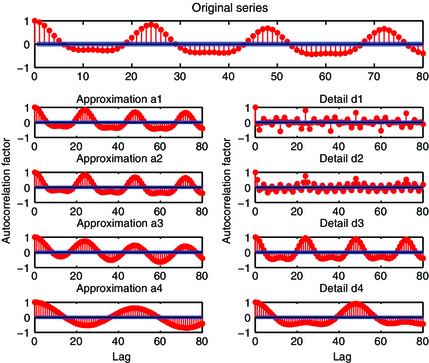Figure 1. The autocorrelation factor (ACF) of original series, approximations (a1, a2, a3, a4) and details (d1, d2, d3, d4).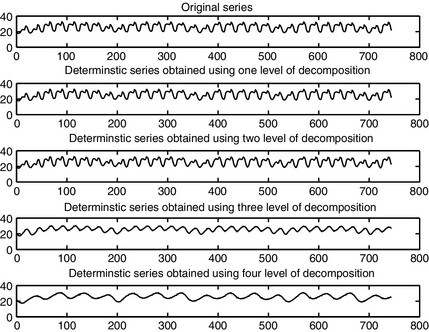Figure 2. The original series and the deterministic series obtained after one, two, three and four levels of decomposition.

### Forecasting process

The proposed methodology is applied to the training data and the 24 h ahead forecast is obtained. The 2-week training window for a particular day is shifted 1 day ahead, and the forecasts for the next 24 h are obtained. In this way, the forecast for the entire year is obtained. This has been carried out to illustrate the accuracy of the proposed model across all seasons and days of the whole year. The performance of the model is evaluated using the mean absolute percentage error (MAPE) metric. The MAPE is computed as follows:

 ${\mbox{MAPE}}={\frac {1}{N}}\sum _{i=1}^{N}{\frac {\vert l_{i}-{\overset {\mbox{ˆ}}{l}}_{i}\vert }{l_{i}}}\times 100$
(11)

where li is the actual load, ${\textstyle {\overset {\mbox{ˆ}}{l}}_{i}}$ is the predicted load and N is the number of predictions. The two variations of MAPE  namely WMAPE (weekly mean absolute percentage error) and MMAPE (monthly mean absolute percentage error) are also used here to evaluate the performance of the proposed model across all months of the year in Spain and California markets. For WMAPE, N = 168 in equation (10) and for MMAPE N = 720 in (11) for months having 30 days, N = 744 for months having 31 days and N = 672 (696) for the month of February (leap year). The illustrations in Figure 1 provide the actual load data and forecast data using the proposed technique in the best and worst forecast weeks of the whole year separately in the two markets. The plots Figure 3A and B provide the best and worst forecast week load corresponding to the California market while Figure 3C and D provide the same for the Spanish market. The accuracy of the forecasts can be seen in the presented plots. The daily MAPE values of the corresponding weeks (best and worst) are provided in Table 1. The MAPE values obtained by the direct application of the WNN technique to the load data are given against the respective best and worst forecast week daily MAPE values under the column (DWNN). The worst daily MAPE values of the proposed model given in the table are obtained during the week of June 8–14, a crucial period for the electricity market of California, while the corresponding values of the Spanish market are obtained during the period December 22–28. The demand (load) values of December 24, 25 has led to this variation in accuracy in the Spain market. The WMAPE values of the best and the worst forecast week in each month of the entire year for the two markets is given in Table 2. From the table, it is observed that the worst forecast weeks are in the months of June and September in the California market while it is June and December for the Spain market reflecting the observations made from Table 1. The accuracy of the proposed model using different wavelets is further illustrated through the MMAPE values presented in Tables 3 and 4.

Table 1. The daily MAPE values of best and worst forecast weeks in California and Spain markets
California market Spain market
Best week Worst week Best week Worst week
DWNN Proposed method DWNN Proposed method DWNN Proposed method DWNN Proposed method
1. MAPE, mean absolute percentage error; DWNN, direct application of weighted nearest neighbor.

Day1 2.8128 1.3887 4.5692 4.2969 0.9515 0.8174 2.8579 1.982
Day2 0.9605 0.6948 1.146 1.1021 0.7738 0.5752 3.5246 3.3518
Day3 0.9384 0.8665 4.4306 2.3888 0.6037 0.4946 8.0344 5.1722
Day4 0.7586 0.6826 4.3076 2.4365 1.0382 0.851 15.3649 9.6267
Day5 0.5688 0.4133 3.1611 3.1611 0.8857 0.6644 3.2774 3.1772
Day6 0.6963 0.6889 5.5658 3.4264 1.5384 1.3081 1.9186 1.2106
Day7 1.4588 0.8554 3.7194 3.6794 1.2972 1.0866 2.3265 1.9288

Table 2. Best and worst WMAPEs for each month of California and Spain markets
Month California market Spain market
Best WMAPE Worst WMAPE Best WMAPE Worst WMAPE
1. WMAPE, weekly mean absolute percentage error.

Jan 0.7986 1.5239 1.1307 2.4124
Feb 0.988 1.3641 1.0142 1.2599
Mar 0.8477 2.1833 1.409 2.6598
Apr 1.3016 2.0315 1.2492 2.8317
May 1.607 2.1965 1.3636 1.9438
June 1.0493 2.9273 1.3094 3.1586
July 1.5524 2.5235 0.8282 1.2873
Aug 1.1746 1.8162 1.411 2.6406
Sep 2.2603 2.764 1.2515 1.4113
Oct 1.3415 2.1079 1.0204 1.5917
Nov 0.8562 2.0543 1.3058 1.9814
Dec 1.0153 1.6756 1.3477 3.7785

Table 3. Monthly mean absolute percentage error (MMAPE) for all the months of California Market in the year 2000
Month NF DWNN Proposed model
db2 db4 db5 Bior 3.1
1. NF, Naïve forecasts; DWNN, direct application of weighted nearest neighbor.

Jan 2.8021 1.491 1.3052 1.3623 1.3272 1.2739
Feb 2.2427 1.4555 1.3447 1.333 1.3158 1.1375
Mar 2.1053 1.3666 1.2204 1.2141 1.1631 1.2108
Apr 3.9983 2.0511 1.7989 1.9053 1.7912 1.7127
May 5.9454 2.2664 2.056 2.0427 2.0286 1.8607
June 6.5311 2.3176 2.0358 2.1026 2.0877 1.8147
July 8.1034 2.3075 2.107 2.1471 2.1388 1.94
Aug 5.9498 2.2675 1.9787 1.877 1.8849 1.6251
Sep 10.1172 3.4761 3.0145 3.1271 3.2 2.4486
Oct 3.8869 2.1147 1.95 1.9127 1.9246 1.7665
Nov 3.9323 1.6718 1.4762 1.5342 1.4972 1.4291
Dec 3.9497 1.596 1.519 1.4691 1.4408 1.2924

Table 4. Monthly mean absolute percentage error (MMAPE) for all the months of Spain market in the year 2002
Month NF DWNN Proposed model
db2 db4 db5 Bior 3.1
1. NF, Naïve forecasts; DWNN, direct application of weighted nearest neighbor.

Jan 4.6634 1.9066 1.5933 1.7835 1.6441 1.5986
Feb 2.2909 1.3265 1.1287 1.1771 1.1415 1.0942
Mar 7.5699 2.4922 2.0219 2.1089 2.1272 1.9568
Apr 5.5462 2.1256 2.0098 2.0344 1.9602 1.8310
May 4.1926 1.8513 1.6543 1.568 1.7094 1.5423
June 4.386 2.3805 1.9297 1.916 1.8864 1.9262
July 2.2819 1.3085 1.1256 1.1407 1.1872 1.0953
Aug 5.9948 2.3159 2.0229 2.0432 2.112 1.9489
Sep 3.12 1.5873 1.4142 1.4662 1.4105 1.2993
Oct 3.0454 1.5125 1.375 1.4337 1.3757 1.2692
Nov 4.848 2.0156 1.7737 1.8468 1.7926 1.6046
Dec 8.1312 2.6973 2.4016 2.4705 2.3662 2.1591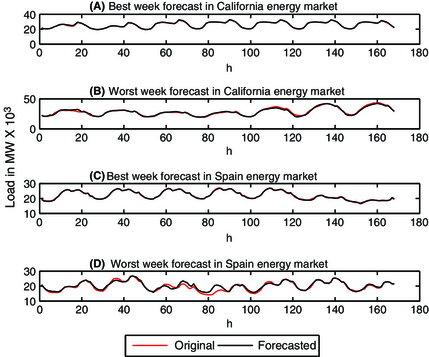Figure 3. The original and forecast load data in the best and worst forecast weeks of California and Spain Markets in the year 2000 and 2002, respectively.

There is no obvious standard model to which the performance of a proposed load forecasting method could be compared . However, to gain some insight into the performance of the model, the results are compared with the Naïve forecasts (NF) and the direct application of the WNN model  on load data. The NF is useful in correctly capturing the shapes of forecasted profiles, but not their scaling . The NF for the loads on day “d” are given by the loads on the day with the same denomination in the week before, d − 7:

 $L(d,\quad h)=L(d-7,\quad h),\quad h=1,\quad 2,\quad \ldots ,\quad 24.$

One day ahead MMAPE for all the months of California market in the year 2000 is given in Table 3. The first column values correspond to the NF values, second column to the nonwavelet application of WNN (DWNN) and the rest of the columns give the measure values corresponding to the proposed model for the wavelets db2, db4, db5 and bior 3.1. From the results, one can observe that bior 3.1 gives the best MMAPE for all the months except March. In the month of March, the data are more skewed and for such a case db5 or db2 gives better results. The corresponding 1 day ahead MMAPE for all the months of the Spain market in the year 2002 is given in Table 4. The wide variations in the climatic conditions of Spain and the significant outliers have resulted in MMAPE values being slightly higher than that of California. Here too, bior 3.1 was able to give the best results for almost all the months of the year. The proposed technique has been evaluated across all the days of the year, irrespective of the seasons and special events/days to determine the adaptability of the wavelet preprocessing techniques to these factors/variations. The results demonstrate that the proposed wavelet-based model is seen to increase the forecast accuracy by 15% to 30% compared to the corresponding nonwavelet model [31, 32].

Prediction behavior of the proposed technique in the best forecast month throughout the year is given in Figure 4 for the California market and in Figure 5 for the Spain market. The closeness of the predicted values of the load with the actual values during the entire month is seen in the figures. Analyzing the results represented in Figures 3-5 and Tables 1-4, it can be concluded that the forecast accuracy of the proposed technique is superior to that of the WNN technique. In other words, the forecast accuracy of WNN technique can be considerably improved by wavelet preprocessing.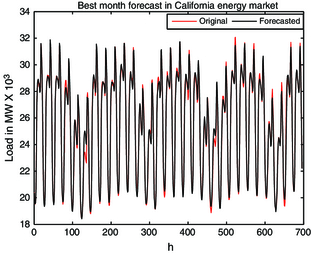Figure 4. The original and forecast load data in the best forecast month of the year 2000 in the California market.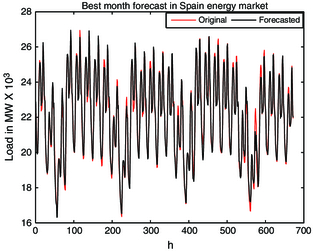Figure 5. The original and forecast load data in the best forecast month of the year 2002 in the Spain market.

## Conclusion

A new load forecasting model based on WT combined with the WNN technique is presented in this paper. The wavelets considered in this study are db2, db4, db5 and bior 3.1. The analysis of the results obtained indicates that the basic idea of the proposed model in reducing the complex structure of electrical load data by splitting it into deterministic and fluctuation components works well as it has considerably improved the performance of the proposed model over the nonwavelet model. Moreover, the model is seen to perform well, irrespective of the seasons and holidays. The Bior 3.1 wavelet is seen to perform well across all the months of the whole year in the energy markets of California and Spain for the years of 2000 and 2002, respectively. The accuracy of the WNN model is increased by around 25% on integrating WT with WNN model.

## Conflict of Interest

None declared.

### Document informationPublished on 01/06/17
Submitted on 01/06/17

Licence: Other

### Document Score0

Views 11
Recommendations 0